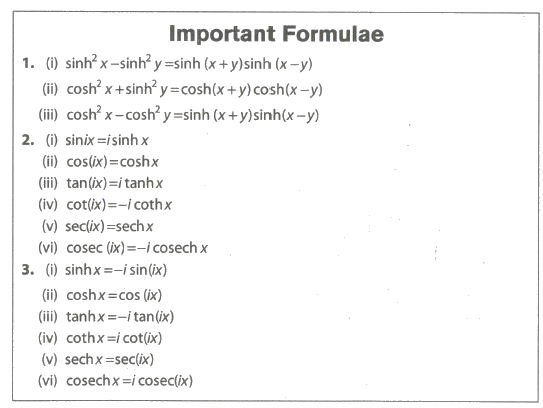# Trigonometric Ratios and Identities Notes Class 11th Maths

0
1868

Angle

When a ray OA starting from its initial position OA rotates about its end point 0 and takes the final position OB, we say that angle
AOB (written as ∠ AOB) has been formed. The amount of rotation from the initial side to the terminal side is Called the measure of
the angle.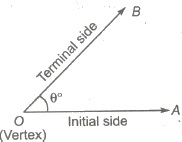Positive and Negative Angles

An angle formed by a rotating ray is said to be positive or negative depending on whether it moves in an anti-clockwise or a clockwise direction, respectively.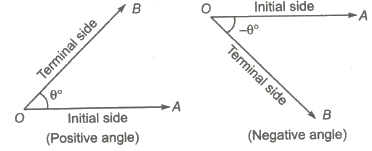Measurement of Angles

There are three system for measuring angles,

1. Sexagesimal System/Degree Measure (English System)

In this system, a right angle is divided into 90 equal parts, called degrees. The symbol 1° is used to denote one degree. Each degree is divided into 60 equal parts, called minutes and one minute is divided into 60 equal parts, called seconds. Symbols 1′ and 1″ are used to denote one minute and one second, respectively.

i.e., 1 right angle = 90°
1° = 60′
1′ = 60″

2. Centesimal System (French System)

In this system, a right angle is divided into 100 equal parts, called ‘grades’. Each grade is subdivided into 100 min and each minute is divided into 100 s.

i.e., 1 right angle = 100 grades = 100g
1g = 100′
1′ = 100″

In this system, angle is measured in radian.

A radian is the angle subtended at the centre of a circle by an arc, whose length is equal to the radius of the circle.

The number of radians in an angle subtended by an arc of circle at the centre is equal to arc/radius.

Relationships

where, π = 22/7 = 3.14159

(iii) If D is the number of degrees, R is the number of radians and G is the number of grades in an angle θ, then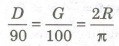(iv) θ = l/r where θ = angle subtended by arc of length / at the centre of the circle, r = radius of the circle.

Trigonometric Ratios

Relation between different sides and angles of a right angled triangle are called trigonometric ratios or T-ratios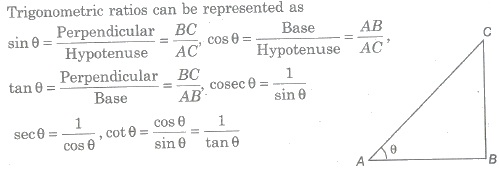Trigonometric (or Circular) Functions

Let X’OX and YOY’ be the coordinate axes. Taking 0 as the centre and a unit radius, draw a circle, cutting the coordinate axes at A,B, A’ and B’, as shown in the figure.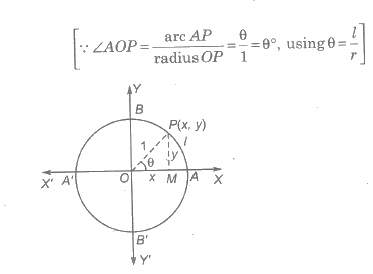Now, the six circular functions may be defined as under
(i) cos θ = x
(ii) sin θ = y
(iii) sec θ = 1/x, x ≠ 0
(iv) cosec θ = 1/y, y ≠ 0
(v) tan θ = y/x, x ≠ 0
(vi) cot θ = x/y, y ≠ 0

Domain and Range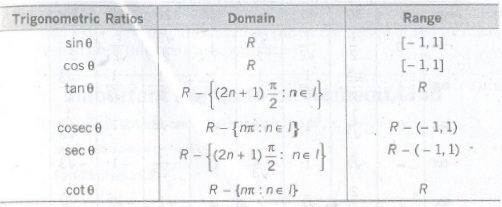Range of Modulus Functions

|sin θ|≤ 1, |cos θ| ≤ 1, |sec θ| ≥ 1, |Cosec θ| ≥ 1 for all values of 0, for which the functions are defined.

Trigonometric Identities

An equation involving trigonometric functions which is true for all those angles for which the functions are defined is called trigonometrical identity. Some identities are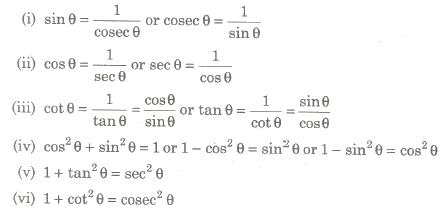Sign of Trigonometric Ratios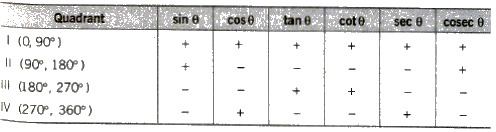Trigonometric Ratios of Some Standard Angles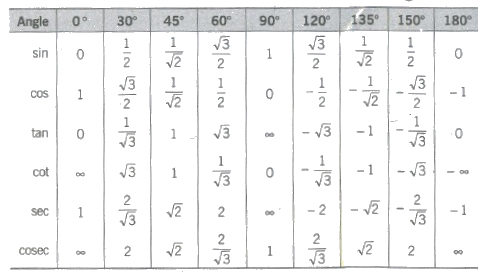Trigonometric Ratios of Some Special Angles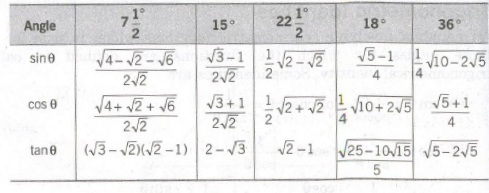Trigonometric Ratios of Allied Angles

Two angles are said to be allied when their sum or difference is either zero or a multiple of 90°. The angles — θ, 90° ± θ, 180° ± θ, 270° + θ, 360° —θ etc., are angles allied to the angle θ, if θ is measured in degrees.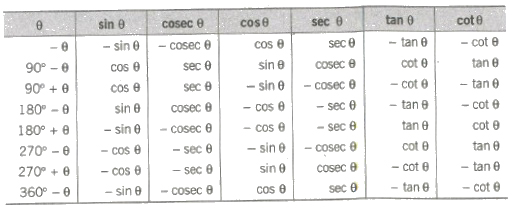Trigonometric Periodic Functions

A function f(x) is said to be periodic, if there exists a real number T> 0 such that f(x + T)= f(x) for all x. T is called the period of the function, all trigonometric functions are periodic.Maximum and Minimum Values of Trigonometric Expressions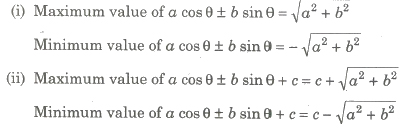Trigonometric Ratios of Compound Angles

The algebraic sum of two or more angles are generally called compound angles and the angles are known as the constituent angle. Some standard formulas of compound angles have been given below.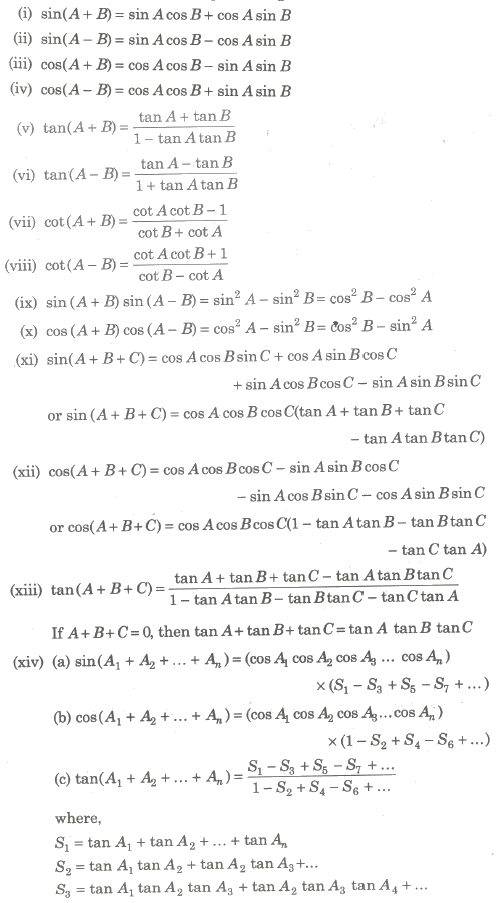Transformation Formulae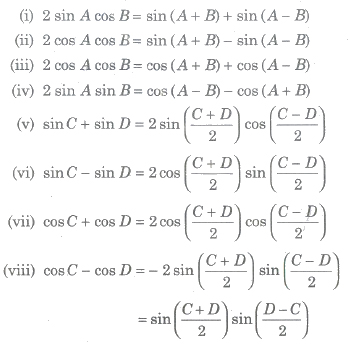Trigonometric Ratios of Multiple Angles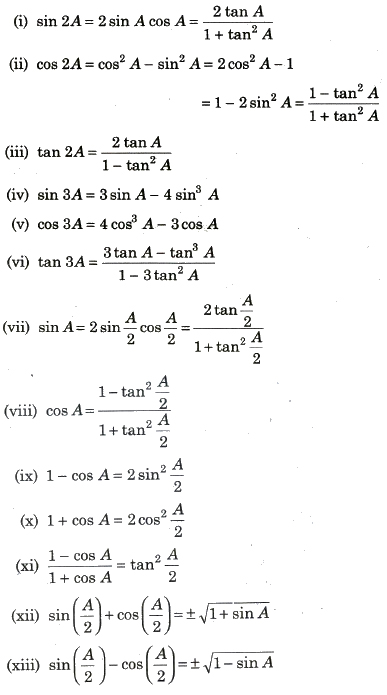Trigonometric Ratios of Some Useful Angles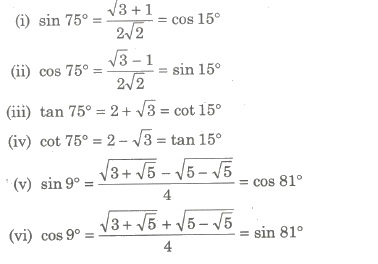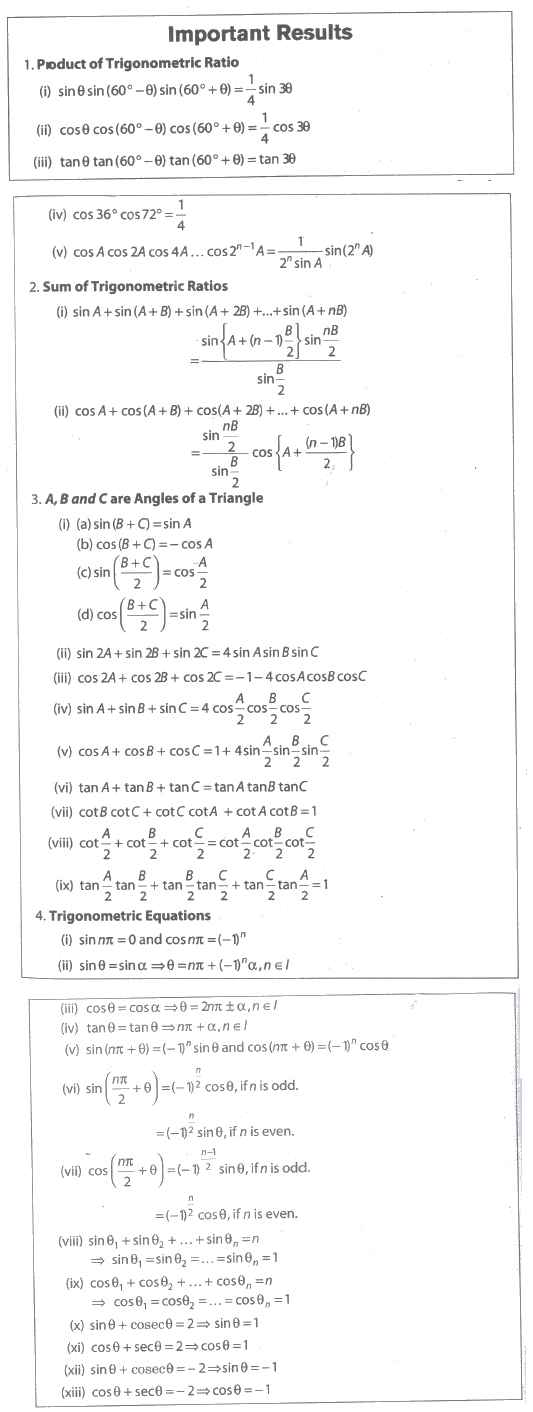Hyperbolic Functions

The hyperbolic functions sinh , cosh z, tanh z, cosech z, sec h z , coth z are angles of the circular functions, defined by removing is appearing in the complex exponentials.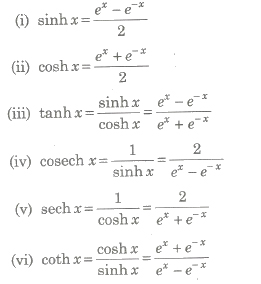Domain and Range of Hyperbolic Function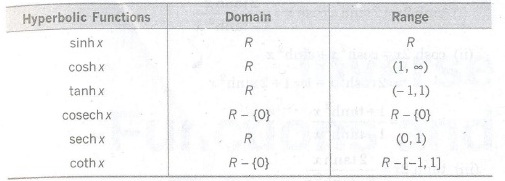identities

(i) cosh2x – sinh2x = 1
(ii) sech2x + tanh2x =1
(iii) coth2x – cosech2x = 1
(iv) cosh2x + sinh2x = cosh2x

Formulae for the Sum and Difference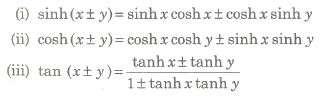Formulae to Transform the Product into Sum or Difference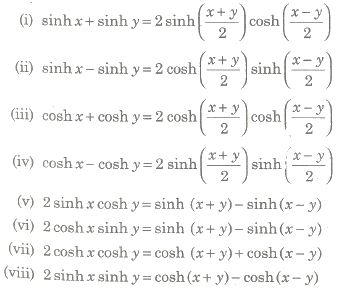Formulae for Multiples of x